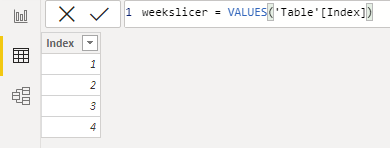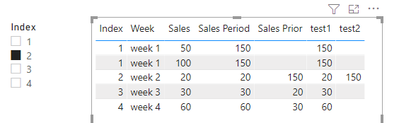cancel
Showing results for
Did you mean:Regular Visitor

## Previous period measure (no date)

Hi community,

I would like to create one simple measure for previous period revenue without using "date" field. So I have created an index for each update I will do (every week). I will use a slicer on the index/week, so if i select week 2, prior should show week 1 figures.

I have several rows so I am using the following formula to calculate "Sales Period" :

Sales Period = SUMX(FILTER(Sheet1, MAX(Sheet1[index])),Sheet1[sales])

How can we calculate "Sales Prior" ? I've tried the following DAX but it's not working :S

Sales Prior = SUMX(FILTER(Sheet1, MAX(Sheet1[index])-1),Sheet1[sales])

 Index Week Sales Sales Period Sales Prior 1 week 1 50 1 week 1 100 2 week 2 20 20 150 3 week 3 30 30 20

1 ACCEPTED SOLUTIONCommunity Support

Hi @EddyBA

You need to create an independent Week table,

``weekslicer = VALUES('Table'[Index])``then create the measures bellow,

``test1 = CALCULATE(SUM('Table'[Sales]),ALLEXCEPT('Table','Table'[Week]))``
``````test2 =
VAR _week =
SELECTEDVALUE ( weekslicer[Index] ) - 1
VAR _valuePrior =
CALCULATE (
SUM ( 'Table'[Sales] ),
FILTER ( ALL ( 'Table' ), 'Table'[Index] = _week )
)
RETURN
IF (
MIN ( 'Table'[Index] ) = SELECTEDVALUE ( weekslicer[Index] ),
_valuePrior,
BLANK ()
)``````Best Regards,

Community Support Team _Tang

If this post helps, please consider Accept it as the solution to help the other members find it more quickly.

4 REPLIES 4Community Support

Hi @EddyBA

You need to create an independent Week table,

``weekslicer = VALUES('Table'[Index])``then create the measures bellow,

``test1 = CALCULATE(SUM('Table'[Sales]),ALLEXCEPT('Table','Table'[Week]))``
``````test2 =
VAR _week =
SELECTEDVALUE ( weekslicer[Index] ) - 1
VAR _valuePrior =
CALCULATE (
SUM ( 'Table'[Sales] ),
FILTER ( ALL ( 'Table' ), 'Table'[Index] = _week )
)
RETURN
IF (
MIN ( 'Table'[Index] ) = SELECTEDVALUE ( weekslicer[Index] ),
_valuePrior,
BLANK ()
)``````Best Regards,

Community Support Team _Tang

If this post helps, please consider Accept it as the solution to help the other members find it more quickly.Super User

Hi,

Have a look at EARLIER function:

https://docs.microsoft.com/fi-fi/dax/earlier-function-dax

I hope this post helps to solve your issue and if it does consider accepting it as a solution and giving the post a thumbs up!

Proud to be a Super User!Regular Visitor

Thank you ValtteriN,

I have already tried this function but unfortunately it's not working.Super User

Hi,

Another option that comes to my mind besides utilizing EARLIER is separating weeknum into its own column and using that in the calculation. e.g.

[earlierWeekSales] =
var _week = MAX(calendar[weeknum])
var _latestweek = CALCULATE(MAX(Table[weeknum]),ALL(calendar),table[weeknum]<_week)
return
CALCULATE(SUM(table[value]),ALL(calendar),table[weeknum]=_latestweek)

Edit: you might need to add years to the calculate, but the basic logic is the same

Proud to be a Super User!Announcements#### Exclusive opportunity for Women!

Join us for a free, hands-on Microsoft workshop led by women trainers for women where you will learn how to build a Dashboard in a Day!#### Power Platform Conference-Power BI and Fabric Sessions

Join us Oct 1 - 6 in Las Vegas for the Microsoft Power Platform Conference.Top Solution Authors
Top Kudoed Authors
Users online (3,835)